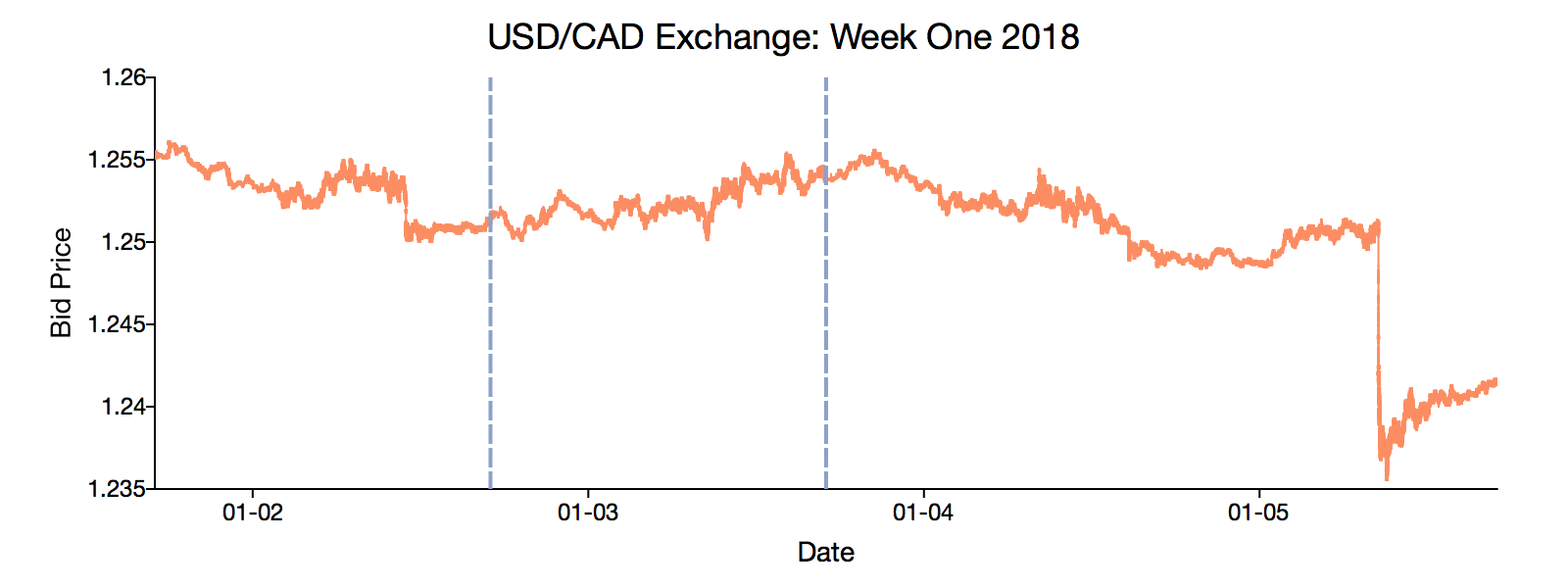# Enhanced date and time management in GAUSS 19## Directly read date and time data using formula strings

// Tell GAUSS that 'RateDateTime' is a string date.
data = loadd("usd_cad_wk1.csv", "date(\$RateDateTime) + RateBid");

The new formula string date operator transforms the way dates and times are read into GAUSS.

• Automatically detects and converts many common date/time formats.
• Supports hundredths of a second and nanoseconds.

## Convert date formats with flexible formatting options

• Easy to use.
• Convert to and from almost any string format.
• Supports DT Scalars and POSIX date/times.

### String date/time to seconds since the Epoch...

dt_posix = strctoposix(str_date, fmt_str);
$\left[\begin{array}{c}\text{"2016-03-28 11:31:43"}\\ \text{"2016-04-01 11:34:27"}\end{array}\right]\stackrel{\text{strctoposix}}{\to }\left[\begin{array}{c}1459164703\\ 1459251267\end{array}\right]$

### ...and back to strings in your preferred format

// Convert to friendly string formats for tables and reports
print posixtostrc(dt_posix, fmt_str2);
$\left[\begin{array}{c}1459164703\\ 1459510467\end{array}\right]\stackrel{\text{posixtostrc}}{\to }\left[\begin{array}{c}\text{"Monday, March 28, 2016"}\\ \text{"Friday, April 1, 2016"}\end{array}\right]$

### DT Scalars to friendly string formats

// Convert DT dates to strings for easy reading
dt_str = dttostrc(dt, "%D");
$\left[\begin{array}{c}\text{"03/17/1912"}\\ \text{"09/04/1937"}\end{array}\right]\stackrel{\text{strctodt}}{\to }\left[\begin{array}{c}19120317060424\\ 19370904010928\end{array}\right]$

### ...and back to DT Scalars

// Convert string dates to DT Scalars for plotting
dt = strctodt(dt_str, "%D");
$\left[\begin{array}{c}\text{"03/17/1912"}\\ \text{"09/04/1937"}\end{array}\right]\stackrel{\text{strctodt}}{\to }\left[\begin{array}{c}19120317060424\\ 19370904010928\end{array}\right]$

## Perform operations on date and time data

GAUSS 19 includes a new suite of time series tools for creating and working with time vectors. Operations on time series data are intuitive and flexible across frequencies:

• Generate date vectors of varying frequency and length.
• Advance or regress date vectors.
• Find differences in dates and times.

### Create sequences of dates

seqadt, shown below, creates sequences of DT scalar dates. Sequences of POSIX dates can be created with seqaposix

// Create a vector starting at January 1980, with
// five elements, each incremented by one month
dt_mths = seqadt(198001, 1, "months", 5);

// Create a vector starting at March 1st, 2016, with
// 6 elements, each incremented by seven days
dt_weeks = seqadt(20160301, 7, "days", 6);

// Create a vector starting at March 1st, 2016 at 09:30:00, with
// 6 elements, each incremented by 12 seconds
dt_sec = seqadt(20160301093000, 12, "seconds", 6);

dt_mths = 19800101000000   dt_weeks = 20160301000000   dt_sec = 20160301093000
19800201000000              20160308000000            20160301093012
19800301000000              20160315000000            20160301093024
19800401000000              20160322000000            20160301093036
19800501000000              20160329000000            20160301093048
20160405000000            20160301093100

// Advance 17 days from July 21, 1984

// Regress 3 days from January 8, 1970
posix_minus = timedeltaposix(604800, -3, "days");

dt_plus = 19840807000000    posix_minus = 345600

### Find the difference between two dates

// How many days between April 16 and July 21, 2012
diff_days = timediffdt(20120416, 20120721, "days");

// How many seconds between 09:38:21 and 09:31:00
diff_sec = timediffdt(20120524093821, 20120524093100,  "seconds");

diff_days = -96    diff_sec = 441